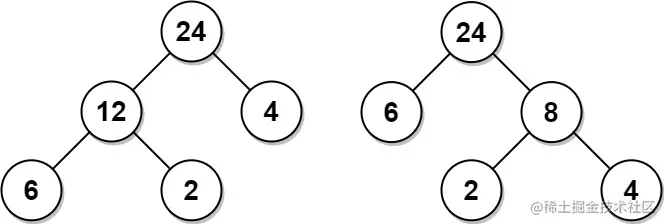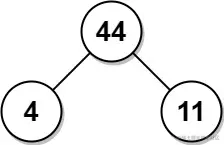# leetcode 1130. Minimum Cost Tree From Leaf Values（python）

2022-02-01 02:00:14

「 This is my participation 11 The fourth of the yuegengwen challenge 21 God , Check out the activity details ：2021 One last more challenge

### describe

Given an array arr of positive integers, consider all binary trees such that:

• Each node has either 0 or 2 children;
• The values of arr correspond to the values of each leaf in an in-order traversal of the tree.
• The value of each non-leaf node is equal to the product of the largest leaf value in its left and right subtree, respectively.

Among all possible binary trees considered, return the smallest possible sum of the values of each non-leaf node. It is guaranteed this sum fits into a 32-bit integer.

A node is a leaf if and only if it has zero children.

Example 1:``````Input: arr = [6,2,4]
Output: 32
Explanation: There are two possible trees shown.
The first has a non-leaf node sum 36, and the second has non-leaf node sum 32.
Copy code ``````

Example 2:``````Input: arr = [4,11]
Output: 44
Copy code ``````

Note:

``````2 <= arr.length <= 40
1 <= arr[i] <= 15
It is guaranteed that the answer fits into a 32-bit signed integer (i.e., it is less than 2^31).
Copy code ``````

### analysis

According to the meaning , Given an array of positive integers arr , Consider all binary trees , bring ：

• Each node has 0 or 2 A child
• arr The value of corresponds to the value of each leaf in the middle order traversal of the tree
• The value of each non leaf node is equal to the product of the maximum leaf value in its left and right subtrees

There may be many kinds of binary trees , The problem requires us to return the minimum possible sum of the values of all non leaf nodes . The title will ensure that the sum is in 32 Bit integer within . A node is a leaf if and only if it has zero children .

First of all, through a simple observation case, we find that , Try to put a large value on the leaf node with shallow depth , Put a small value on the deeper leaf node , In this way, the result of the final multiplication will be as small as possible . There are generally three situations , Let's give three simple examples ：

• Example a ：[1,2,3] , The method of multiplication is 1 and 2 Take it first 2 , then 2 and 3 Multiply , Operate from left to right
• Example 2 ：[3,2,1] , The method of multiplication is 1 and 2 Take it first 2 , then 2 and 3 Multiply , That is, operate from right to left
• Example 3 ：[3,1,2] , take 3 and 2 The smaller value of is 2 , First pair 1 and 2 By multiplication 2 , Again 2 and 3 Multiply

Because the limit of value in the title is 1 To 15 Between , Add an extra large value before example 1 100 , Just like example 3, the law is the same , So there are two situations . We maintain a stack stack Add an extra large value at the beginning 100 , First calculate the product sum according to the logic of example 3 , Then calculate the product sum according to the logic of example 2 .

``````class Solution(object):
def mctFromLeafValues(self, arr):
"""
:type arr: List[int]
:rtype: int
"""
stack = 
result = 0
for current in arr:
while stack[-1]<=current:
drop = stack.pop()
result += drop*(min(stack[-1], current))
stack.append(current)
while len(stack)>2:
result += stack.pop() * stack[-1]
return result

Copy code ``````

### Running results

``````Runtime: 28 ms, faster than 46.53% of Python online submissions for Minimum Cost Tree From Leaf Values.
Memory Usage: 13.4 MB, less than 74.26% of Python online submissions for Minimum Cost Tree From Leaf Values.
Copy code ``````